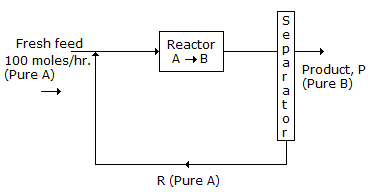# Chemical Engineering - Stoichiometry

### Exercise :: Stoichiometry - Section 5

31.

In a chemical process, the recycle stream is purged for

 A. increasing the product yield. B. enriching the product. C. limiting the inerts. D. heat conservation.

Explanation:

No answer description available for this question. Let us discuss.

32.

The viscosity of water at room temperature may be around one

 A. centipoise B. poise C. stoke D. both (b) &(c)

Explanation:

No answer description available for this question. Let us discuss.

33.

A flowsheet is given in the following figure:If the single pass once through conversion of A to B is 20%, then the rate of recycle R (molds/hr) is

 A. 300 B. 400 C. 500 D. 600

Explanation:

No answer description available for this question. Let us discuss.

34.

The heat capacity of most substances is greater for the __________ state.

 A. solid B. liquid C. gaseous D. none of these

Explanation:

No answer description available for this question. Let us discuss.

35.

The temperature at which real gases obey the ideal gas law over a wide range of pressure is called the __________ temperature.

 A. reduced B. Boyle C. critical D. inversion